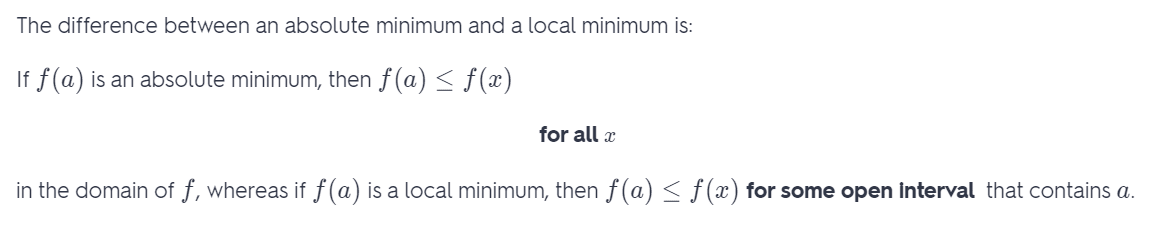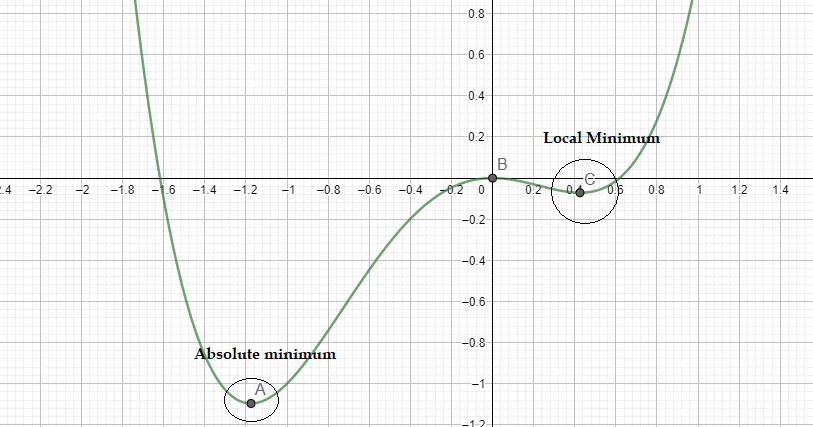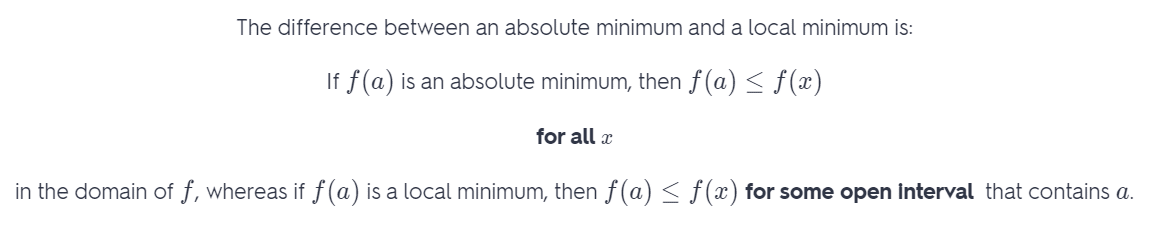# Explain The Difference Between An Absolute Minimum And A Local Minimum.

We thoroughly check each answer to a question to provide you with the most correct answers. Found a mistake? Let us know about it through the REPORT button at the bottom of the page.

Explain the difference between an absolute minimum and a local minimum.

Contents

Local minimum is the smallest value that a function has on some open interval (which is part of domain).

Absolute minimum is the smallest value that a function has on its entire domain.

Note:

Local minimum can be reached in just one point.

There is just one absolute minimum, but it can be reached in multiple point.For example: f(x) = x^4 + x^3 – x^2

A point A is an absolute minimum, also A is local minimum.

A point C is only a local minimum.

The points that are at the end of the graph can never be the local minimum, because there is no open interval around the end point.## Result

Local minimum is the smallest value that a function has on some open interval (which is part of domain).

Absolute minimum is the smallest value that a function has on its entire domain.